Refer to our Texas Go Math Grade 6 Answer Key Pdf to score good marks in the exams. Test yourself by practicing the problems from Texas Go Math Grade 6 Lesson 12.2 Answer Key Addition and Subtraction Equations.

Modeling Equations

A puppy weighed 6 ounces at birth. After two weeks, the puppy weighed 14 ounces. How much weight did the puppy gain?
Let x represent the number of ounces gained.To answer this question, you can solve the equation 6 + x = 14.
Algebra tiles can model some equations. An equation mat represents the two sides of an equation. To solve the equation, remove the same number of tiles from both sides of the mat until the x tile is by itself on one side.
A. Model 6 + x = 14.B. How many 1 tiles must you remove on the left side so that the x tile is by itself? ___________ Cross out these tiles on the equation mat.
C. Whenever you remove tiles from one side of the mat, you must remove the same number of tiles from the other side of the mat. Cross out the tiles that should be removed on the right side of the mat.
D. How many tiles remain on the right side of the mat? ____________ This is the solution of the equation.
The puppy gained ____________ ounces.

Reflect

Question 1.
Communicate Mathematical Ideas How do you know when the model shows the final solution? How do you read the solution?
The model shows final solution when only the variable is left in one side of the mat and the the same number of tiles have been removed. Thus, the remaining tiles on the right side of the mat are 12 which indicates that the puppy gained 12 ounces.

Example 1

Solve the equation a + 15 = 26. Graph the solution on a number line.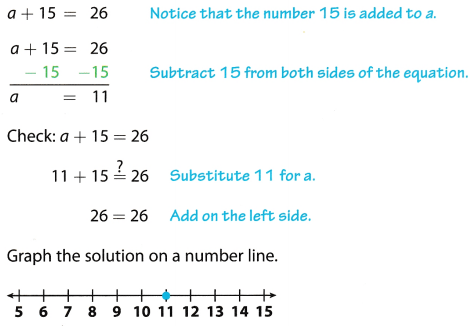Reflect

Question 2.
Communicate Mathematical Ideas How do you decide which number to subtract from both sides?
The reason for solving an equation is to determine the value of the variable on which the equation holds true. For this purpose, the variable is isolated on 1 side of the equation. Therefore, the number associated with the variable on the variable side of the equation is subtracted (or added as required) from both sides of the equation.

Question 3.
Solve the equation 5 = w + 1.5.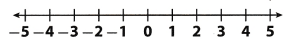Graph the solution on a number line.
w = ____________
Solution to this example is given below
5 = w + 1.5
5 = w + 1.5 Notice that 15 is added tow
5 – 1.5 = w + 1.5 – 1.5 Subtract both sides of the equation by 15
w = 3.5 Simplify
Check; 5 = w + 1.5
5 = 3.5 + 1.5 Substitute 3.5 for w
5 = 5 Add on the right side.
w = 3.5 Final solution
Graph the solution on a number line.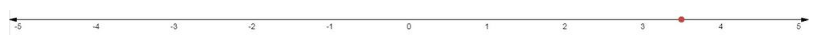Example 2

Solve the equation y – 21 = 18. Graph the solution on a number line.Reflect

Question 4.
Communicate Mathematical Ideas How do you know whether to add on both sides or subtract on both sides when solving an equation?
The reason for solving an equation is to determine the value of the variable on which the equation holds true. For this purpose, the variable is isolated on 1 side of the equation. Therefore, the number associated with the variable on the variable side of the equation is subtracted if its sign is positive or added if its sign is negative from both sides of the equation.

Question 5.
Solve the equation h – $$\frac{1}{2}$$ = $$\frac{3}{4}$$.
Graph the solution on a number line.h = ___________
Solution to this example is given below.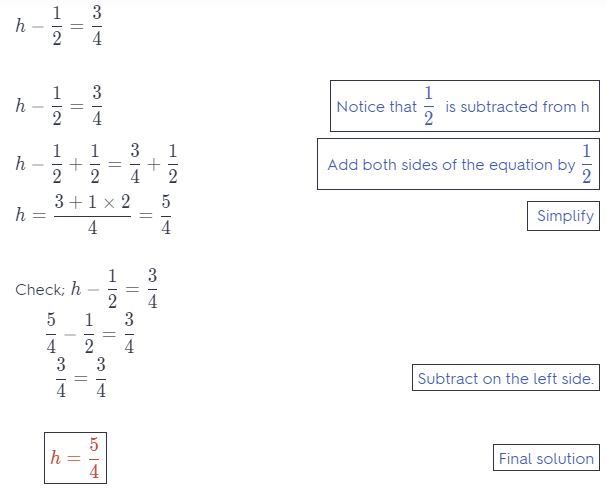Graph the solution on a number line.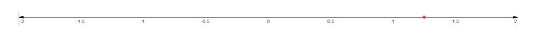Example 3

Find the measure of the unknown angle.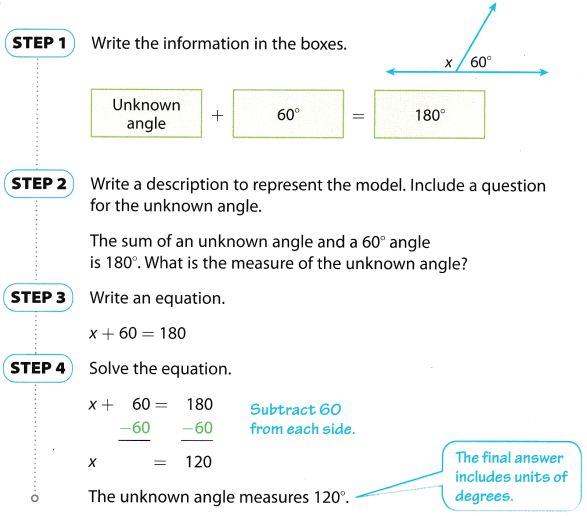Question 6.
Write and solve an equation to find the measure of the unknown angle.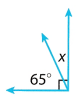It can be seen that the 2 angles are complementary angles so the equation becomes:
x + 65° = 90°
Add 65° on both sides of the equation to isolate the variable on 1 side of the equation:
x + 65° – 65° = 90° – 65°
Evaluate the variable:
x = 25°

Question 7.
Write and solve an equation to find the measure of a complement of an angle that measures 42°.
The sum of a unknown angle and a 42° angle is 90°. What is the measure of the unknown angle?
Write an equation
x + 42° = 90°
x + 42° – 42° = 90° – 42° Subtract 42 from both sides
x = 48° Simplify
x = 48° Final solution
The unknown angle measures 48°

Example 4

Write a real-world problem for the equation 21.79 + x = 25. Then solve the equation.
21.79 + x = 25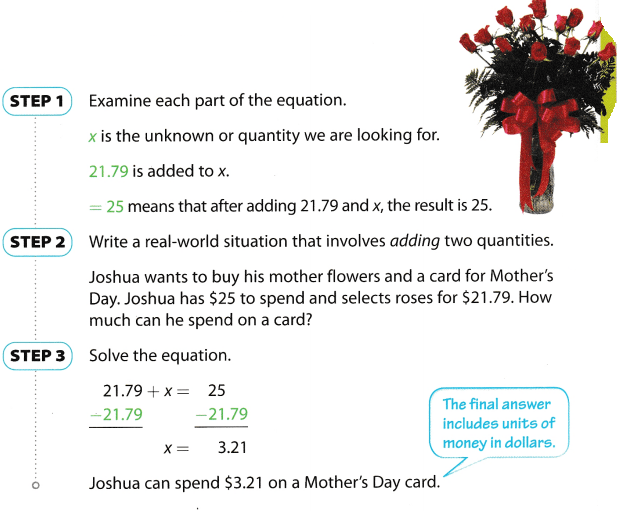Reflect

Question 8.
What If? How might the real-world problem change if the equation were x – 21.79 = 25 and Joshua still spent $21.79 on roses? Answer: In the context of the example, r w the amount to be spent on the card. Here it represents tile total budget. as when this equation is solved: r = 25 + 21.79 =$46.79 and the situation can be to evaluate the total amount such that there should be $25 left to spend on a gift after spending$21.79 on roses.

Question 9.
Write a real-world problem for the equation x – 100 = 40. Then solve the equation.
A real world situation to model the given equation can be the total amount required to he saved, if 100 are in savings and still $40 more are required. Solve the equation: x = 100 + 40 = 140. x = 140 Texas Go Math Grade 6 Lesson 12.2 Guided Practice Answer Key Question 1. A total of 14 guests attended a birthday party. Three guests stayed after the party to help clean up. How many guests left when the party ended? (Explore Activity) a. Let x represent the _____________ Answer: The variable x represents the number of guests who left the party when it ended. b.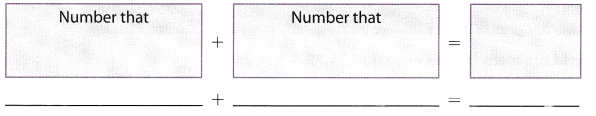Answer: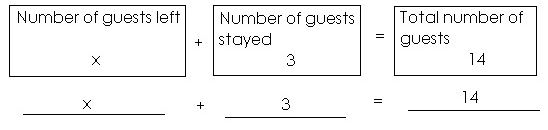c. Draw algebra tiles to model the equation. _________ friends left when the party ended.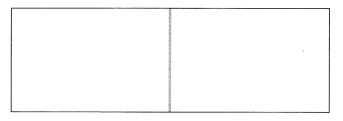Answer: There are 11 friends left when the party ended.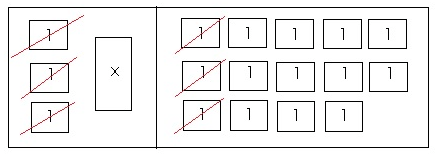Solve each equation. Graph the solution on a number line. (Examples 1 and 2) Question 2. 2 = x – 3 x = ____________Answer: Solution to this example is given below 2 = x – 3 2 = x – 3 Notice that 3 is subtracted from x 2 + 3 = x – 3 + 3 Add both sides of the equation by 3 x = 5 Switch sides Check; 2 = x – 3 2 = 5 – 3 Substitute 5 for x 2 = 2 Subtract on the right side. x = 5 Final solution Graph the solution on a number line.Question 3. s + 12.5 = 14 s = ___________Answer: Solution to this example is given below s + 12.5 = 14 s + 12.5 = 14 Notice that 12.5 is added to s s + 12.5 – 12.5 = 14 – 12.5 Subtract both sides of the equation by 125 s = 1.5 Simplify Check; s + 12.5 = 14 1.5 + 12.5 = 14 Substitute 1.5 for s 14 = 14 Add on the left side. s = 1.5 Final solution Graph the solution on a number line.Question 4. h + 6.9 = 11.4 h = _________ Answer: Solution to this example is given below h + 6.9 = 11.4 h + 6.9 = 11.4 Notice that 6.9 is added to h h + 6.9 6.9 = 11.4 – 6.9 Subtract both sides of the equation by 6.9 h = 4.5 Simplify Check; h + 6.9 = 11.4 4.5 + 6.9 = 11.4 Substitute 4.5 for h 11.4 = 11.4 Add on the Left side. h = 4.5 Final solution h = 4.5 Question 5. 82 + p = 122 p = ____________ Answer: Given equation: 82 + p = 122 Add 82 on both sides of the equation to isolate the variable on 1 side of the equation: 82 + p – 82 = 122 – 82 Evaluate the variable: p = 40 Question 6. n + $$\frac{1}{2}$$ = $$\frac{7}{4}$$ n = ___________ Answer: Given equation: n + $$\frac{1}{2}$$ = $$\frac{7}{4}$$ Add –$$\frac{1}{2}$$ on both sides of the equation to isolate the variable on 1 side of the equation: n + $$\frac{1}{2}$$ – $$\frac{1}{2}$$ = $$\frac{7}{4}$$ – $$\frac{1}{2}$$ Evaluate the variable using Least common denominator technique: n = $$\frac{7-1 \times 2}{4}$$ Simplify: n = $$\frac{7-2}{4}=\frac{5}{4}$$ n = $$\frac{5}{4}$$ Question 7. Write and solve an equation to find the measure of the unknown angle. (Example 3)Answer: It can be seen that the 2 angles are supplementary angles so the equation becomes: x + 45° = 180° Add -45° on both sides of the equation to isolate the variable on 1 side of the equation: x + 45° – 45° = 180° – 45° Evaluate the variable: x = 135° Question 8. Write a real-world problem for the equation x – 75 = 200. Then solve the equation. (Example 4) Answer: John needs to save a certain amount of money for a new bike. He has already saved$75. If he requires $200 more. how much does he need to save? Equation: x – 75 = 200, therefore x = 200 + 75 = 275 Essential Question Check-In Question 9. How do you solve equations that contain addition or subtraction? Answer: Case 1. If the sign of the constant associated with the variable on the variable side of the equation is positive, then the constant is subtracted from both sides of the equation, to isolate the variable on 1 side of the equation. For example: x + 5 = 12 is soLved by subtracting 5 from both sides of the equation, therefore the intermediate step becomes: x + 5 – 5 = 12 – 5 and the solution is x = 7. Case 2. If the sign of the constant associated with the variable on the variable side of the equation is negative, then the constant is added to both sides of the equation, to isolate the variable on 1 side of the equation. For example: x – 5 = 12 is soLved by adding 5 to both sides of the equation, therefore the intermediate step becomes: x – 5 + 5 = 12 + 5 and the solution is x = 17. Texas Go Math Grade 6 Lesson 12.2 Independent Practice Answer Key Write and solve an equation to answer each question. Question 10. A wildlife reserve had 8 elephant calves born during the summer and now has 31 total elephants. How many elephants were in the reserve before summer began? Answer: Solution to this example is given below x + 8 = 31 x + 8 = 31 Notice that 8 ¡s added to x x + 8 – 8 = 31 – 8 Subtract both sides of the equation by 8 x = 23 Simplify Check; x + 8 = 31 23 + 8 = 31 Substitute 23 for x 31 = 31 Add on the left side. x = 23 Final solution There were 23 elephants in the reserve before summer began. Question 11. My sister is 14 years old. My brother says that his age minus twelve is equal to my sister’s age. How old is my brother? Answer: Let the brother’s age be x, then the equation of this age is: x – 12 = 14 Add 12 on both sides of the equation to isolate the variable on 1 side of the equation: x – 12 + 12 = 14 + 12 Evaluate the variable: x = 26 The brother is 26 years old. Question 12. Kim bought a poster that cost$8.95 and some colored pencils. The total cost was $21.35. How much did the colored pencils cost? Answer: Solution to this example is given below x + 8.95 = 21.35 x + 8.95 = 21.35 Notice that 895 is added to x x + 8.95 8.95 = 21.35 – 8.95 Subtract both sides of the equation by 8.95 x = 12.4 Simplify Check; x + 8.95 = 21.35 12.4 + 8.95 = 21.35 Substitute 12.4 for x 21.35 = 21.35 Add on the left side. x = 12.4 Final solution The colored pencils costed 12.4 dollars. Question 13. The Acme Car Company sold 37 vehicles in June. How many compact cars were sold in June?Answer: Let the number of compact cars were sold in Junes be x, then the equation of total cars sold is: x + 8 = 37 Add -8 on both sides of the equation to isoLate the variable on 1 side of the equation: x + 8 – 8 = 37 – 8 EvaLuate the variable: x = 29 29 compact cars were sold in June. Question 14. Sandra wants to buy a new MP3 player that is on sale for$95. She has saved $73. How much more money does she need? Answer: Solution to this example is given below x + 73 = 95 x + 73 = 95 Notice that 73 is added to x X + 73 – 73 = 95 – 73 Subtract both sides of the equation by 73 x = 22 Simplify Check; x + 73 = 95 22 + 73 = 95 Substitute 22 for x 95 = 95 Add on the left side x = 22 Final solution 22 more are required. Question 15. Ronald spent$123.45 on school clothes. He counted his money and discovered that he had $36.55 left. How much money did he originally have? Answer: Let the total amount of money be x, then the equation of total cost is: x = 123.45 + 36.55 Evaluate the variable: x = 160 He originally had$160.

Question 16.
Brita withdrew $225 from her bank account. After her withdrawal, there was$548 left in Brita’s account. How much money did Brita have in her account before the withdrawal?
Let the total amount of money be x, then the equation of money after withdrawal is:
x – 225 = 548
Add 225 on both side of the equation to isolate the variable on 1 side of the equation:
x – 225 + 225 = 548 + 225
Evaluate the variable:
x = 773
There were $773 in the account before the withdrawal. Question 17. Represent Real-World Problems Write a real-world situation that can be represented by 15 + c = 17.50. Then solve the equation and describe what your answer represents for the problem situation. Answer: Solution to this example is given below 15 + c = 17.50 15 + c = 17.50 Notice that 15 is added to c 15 + c – 15 = 17.50 – 15 Subtract both sides of the equation by 15 c = 2.5 Simplify Check; 15 + c = 17.50 15 + 2.5 = 17.50 Substitute 25 for c 17.50 = 17.50 Add on the left side c = 2.5 Final solution Here c = 2.5 is the amount in dollars that can be spent on the card. Question 18. Critique Reasoning Paula solved the equation 7 + x = 10 and got 17, but she is not certain if she got the correct answer. How could you explain Paula’s mistake to her? Answer: Given equation: 7 + x = 10 Add -7 on both sides of the equation to isolate the variable on 1 side of the equation: 7 + x – 7 = 10 – 7 Evaluate the variable: x = 3 Paula added 7 to both sides of the equation instead of adding -7. H.O.T. Focus on Higher Order Thinking Question 19. Multistep Handy Dandy Grocery is having a sale this week. If you buy a 5-pound bag of apples for the regular price, you can get another bag for$1.49. If you buy a 5-pound bag of oranges at the regular price, you can get another bag for $2.49.a. Write an equation to find the discount for each situation using a for apples and r for oranges. Answer: The equation to find the discount for each situation using a for the amount of the discount for apples is a + 1.49 = 2.99 and r for the amount of the discount for oranges is r + 2.49 = 3.99 Solve equation 1: a = 2.99 – 1.49 = 1.5 Solve equation 2: r = 3.99 – 2.49 = 1.5 b. Which fruit has a greater discount? Explain. Answer: It can be seen that the discount in both cases is same and equal to$1.50.

Question 20.
Critical Thinking An orchestra has twice as many woodwind instruments as brass instruments. There are a total of 150 brass and woodwind instruments.
a. Write two different addition equations that describe this situation. Use w for woodwinds and b for brass.
Let w be the number of woodwinds instruments and b for brass instruments, then according to the first situation given: w = 2b. The second equation is w + b = 150
Substitute the value of w = 2b:
2b + b = 150
Simplify:
3b = 150
Divide both sides of the equation with 3 to evaluate the variable:
b = $$\frac{150}{3}$$ = 50

b. How many woodwinds and how many brass instruments satisfy the given information?
There are 50 brass instruments and 2(50) = 100 woodwinds instruments.

Question 21.
Look for a Pattern Assume the following: a + 1 = 2, b + 10 = 20, c + 100 = 200, d + 1,000 = 2,000,…
a. Solve each equation for each variable.
a + 1 – 1 = 2 – 1 subtraction property of equality
a = 1 value of a
b + 10 – 10 = 20 – 10 subtraction property of equality
b = 10 value of b
c + 100 – 100 = 200 – 100 subtraction property of equality
c = 100 value of c
d + 1,000 – 1, 000 = 2,000 – 1,000 subtraction property of equality
d = 1,000 value of d

b. What pattern do you notice between the variables?
The pattern between variables is increasing by multiples of 10.

c. What would be the value of g if the pattern continues?